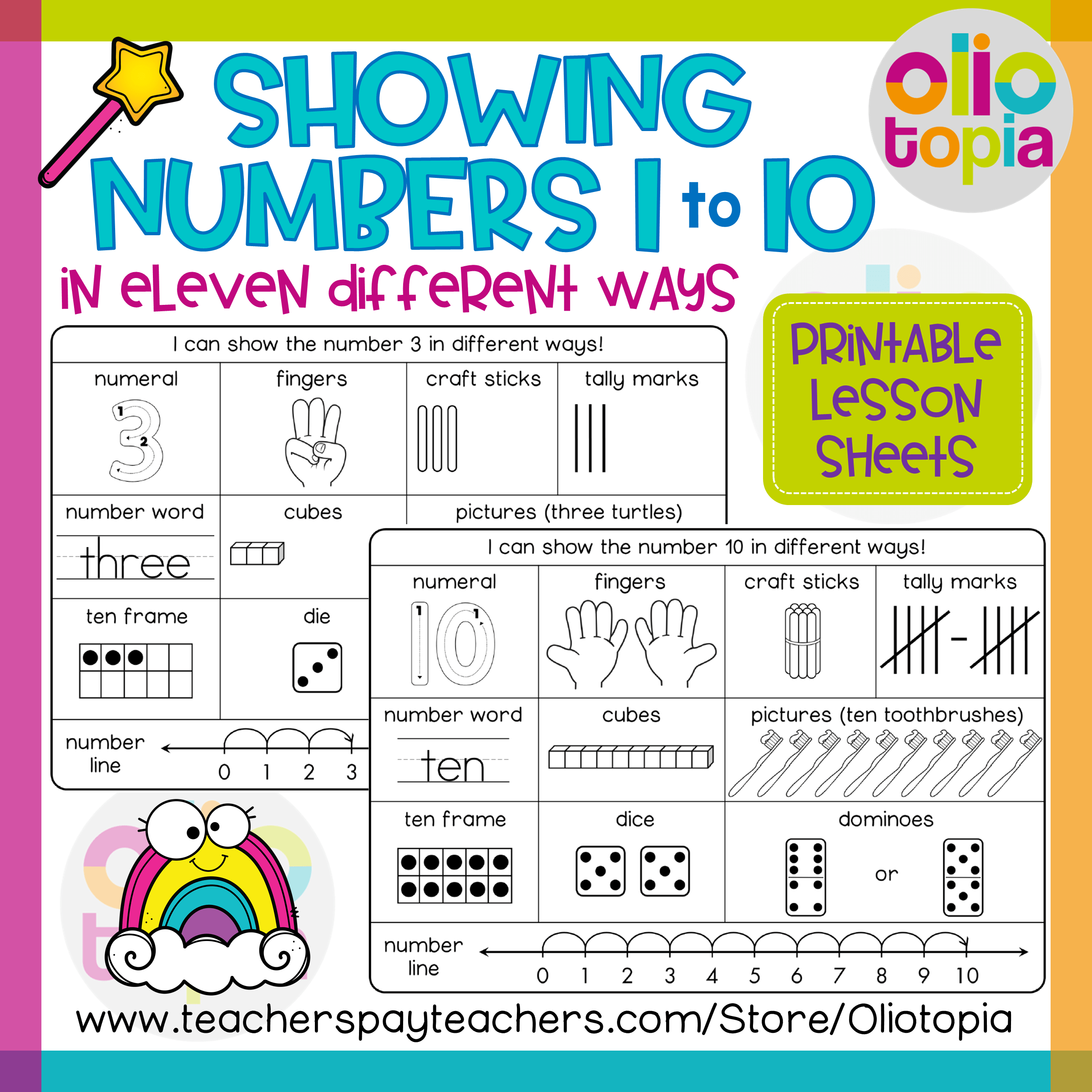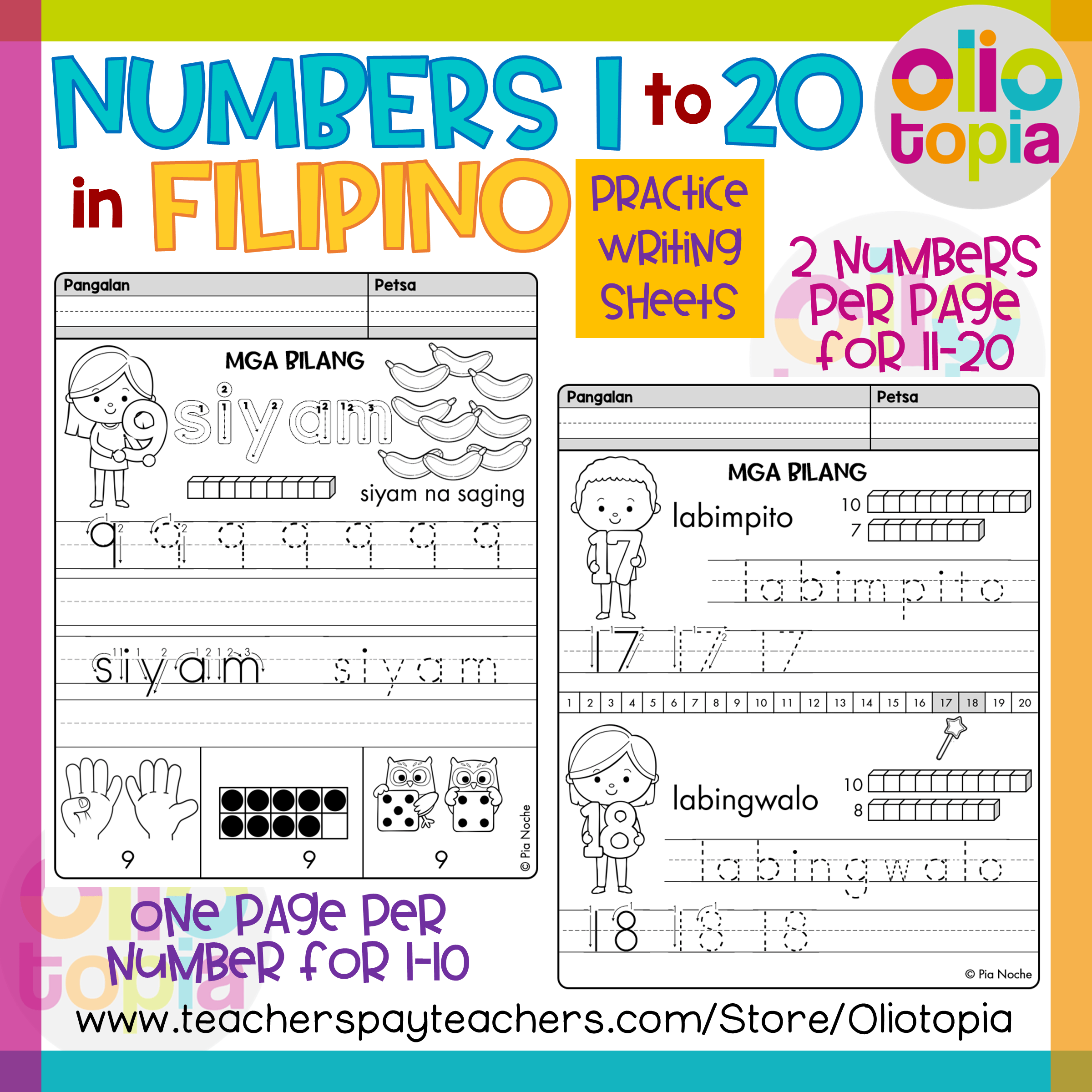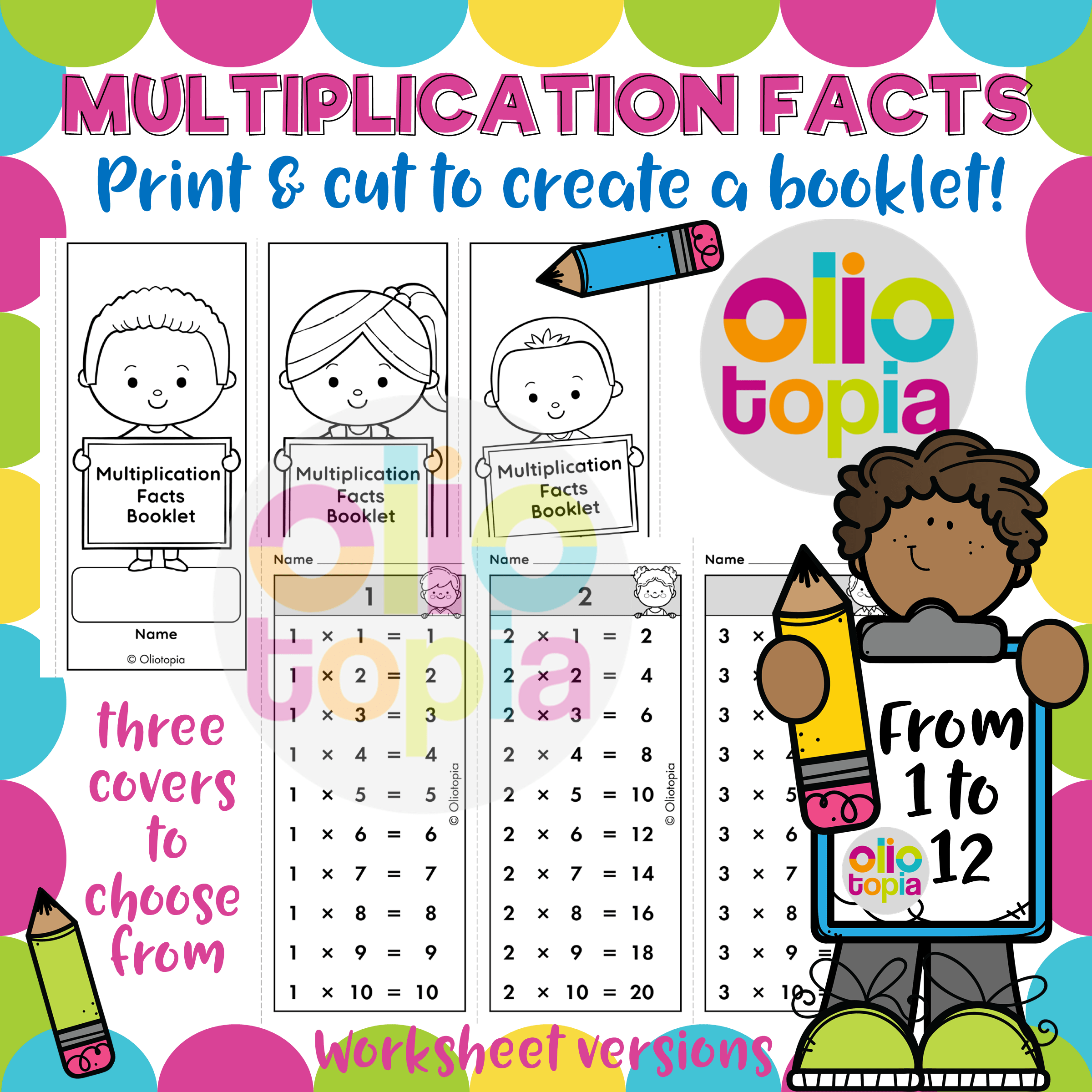Oliotopia | Math

Below are the descriptions of the Math products at my Teachers Pay Teachers store Oliotopia. If you would like to purchase any of the products, email me using the Contact form in the main menu above. U.S. dollar prices will be converted to Philippine pesos.

Showing Numbers 1 to 10 in Different Ways (U.S. \$3.00)Showing Numbers 1 to 10 in Different Ways | 34 pages | \$3.00

Description: This product is written in the English language. It includes the following PDF files described below with a total of 34 pages.

A. Numbers 1-10.pdf: A set of lesson sheets with a page for each number from 1 to 10 showing the number in eleven ways:

1. numeral

2. number word

3. fingers on two hands

4. cubes or base-10 blocks

5. craft sticks

6. tally marks

7. dots in a ten frame

8. die or dice

9. domino or dominoes

10. number line

11. pictures of objects

B. Numbers in Different Ways.pdf: A set of lesson sheets with 4 pages with the numbers 1 to 10 shown as cubes/base-10 blocks, ten frames, tally marks, craft sticks, dice, dominoes, and number lines.

C. Showing Numbers Worksheets.pdf: (1) A set of 11 worksheets (one for each number from 1 to 10 and a blank worksheet) for learners to fill in the different ways to show the given number. (2) Nine worksheets where learners are asked to draw the different representations of numbers 1 to 10 or match the number to a representation. The pages in this product are designed to be printed on letter-sized papers (8.5” x 11”).

Click here to see a preview of “Showing Numbers 1 to 10 in Different Ways”.

Showing Filipino Numbers 1-10 in Different Ways (U.S. \$3.00)Showing Filipino Numbers 1 to 10 in Different Ways | 25 pages | \$3.00

Description: This product is written in the Filipino language. It includes the following:

A. A set of handouts for each number from 1 to 10 showing the number in eleven ways:

1. numeral

2. number word (salitang bilang)

3. fingers on two hands (mga daliri)

4. cubes or base-10 blocks (mga kubo)

5. craft sticks (mga patpat)

6. tally marks (mga markang paya)

7. dots in a ten frame (kuwadro ng sampu)

8. dice (dais)

9. domino

10. number line (linya ng numero)

11. a picture of objects (larawan)

B. A set of handouts with 4 pages with the numbers 1 to 10 shown as base-10 blocks, ten frames, tally marks, craft sticks, dice, dominoes, and number lines.

C. A set of 11 worksheets (one for each number from 1 to 10 and a blank one) for learners to fill in the different ways to show the given number.

The handouts and worksheets in this product are designed to be printed on letter-sized papers (8.5” x 11”). Please download and read my Terms of Use before purchasing this product.

Click here to see a preview of “Showing Filipino Numbers 1-10 in Different Ways”.

Numbers 1 to 20 in Filipino (U.S. \$4.00)Numbers 1 to 20 in Filipino | 31 pages | \$4.00

Description: This product is written in the Filipino language. It includes the following:

1. Practice writing pages on the numerals and number words for numbers 1 to 20. One page for each of the numbers from 1 to 10 and two numbers per page for numbers 11 to 20. Numbers are shown as base 10 blocks, fingers on hands, ten frame, and dice.
2. A set of 14 worksheets for recognizing, ordering, and writing the numbers 1 to 20
3. Two number puzzles for numbers 1 to 10 and 11 to 20. These can be printed and cut into strips. Learners can complete the puzzle by placing the numbers in the correct order to reveal a complete picture.
4. An introduction to the use of Filipino prefixes for numbers 11 to 19.

Click here to see a preview of “Numbers 11-20 in Filipino”.

Multiplication Facts and Chart (U.S. \$3.50)Multiplication Facts and Chart | 45 pages | \$3.50

Description: This 45-page product is written in the English language. It is a set of printable PDF files in black and white. There are 33 pages of lesson sheets and worksheets, and 12 pages of answer keys. The PDF files are designed to be printed on short-sized paper (8.5” x 11”). The zip file includes the following PDF files:

1. Multiplication Facts.pdf (6 pages): A PDF file with multiplication fact tables (3 per page) from 1 to 12 and one table showing the same factors (i.e., 1 x 1, 2 x 2, etc.). A page can be cut into three multiplication tables and made into a booklet. Also included are three versions of a cover page for the booklet.

2. Multiplication Facts_Blanks.pdf (6 pages total): This PDF file is the same as the Multiplication Facts.pdf except that it has blanks for the products. The learner would be able to write the answers for each multiplication fact table from 1 to 12 and a table with similar factors.

3. Multiplication Chart.pdf (5 pages): Includes (a) two versions of the multiplication chart from 1-10, (b) two versions of the multiplication chart from 1 to 12, and (c) a 1-page explanation on how to use the multiplication chart.

4. Complete the Multiplication Chart.pdf (5 pages total): Includes (a) two multiplication charts from 1 to 10 with 50 missing products, (b) two multiplication charts from 1 to 10 with 75 missing products, and (c) one blank multiplication chart from 1-10.

5. Multiplication Facts Shuffled per Number.pdf (4 pages total): Shows multiplication facts tables from 1 to 12 where the facts in each table are not in order.

6. Multiplication Facts 1-3 Shuffled.pdf (1 page): Contains a worksheet showing the multiplication facts for 1, 2, and 3 where the facts are shuffled. The learner can write the products.

7. Multiplication Facts 4-6 Shuffled.pdf (1 page): Contains a worksheet showing the multiplication facts for 4, 5, and 6 where the facts are shuffled. The learner can write the products.

8. Multiplication Facts 7-9 Shuffled.pdf (1 page): Contains a worksheet showing the multiplication facts for 7, 8, and 9 where the facts are shuffled. The learner can write the products.

9. Multiplication Facts 10-12 Shuffled.pdf (2 pages): Contains a worksheet showing the multiplication facts for 10, 11, and 12 where the facts are shuffled. An additional multiplication fact table for similar factors is added. The learner can write the products.

10. Multiplication Facts Mixed Practice.pdf (2 pages): Includes (a) one worksheet with mixed multiplication facts problems for 1 to 5, and (b) one worksheet with mixed multiplication facts problems for 6 to 10.

The rest of the files are the answer keys to the worksheets.

If there are any errors in the product, feel free to email me at oliotopia@gmail.com and I will make the necessary corrections.

Once you have purchased this product, it is understood that you agree to abide by the Terms of Use of this TpT store.

Click here to see a preview of “Multiplication Facts and Chart”.